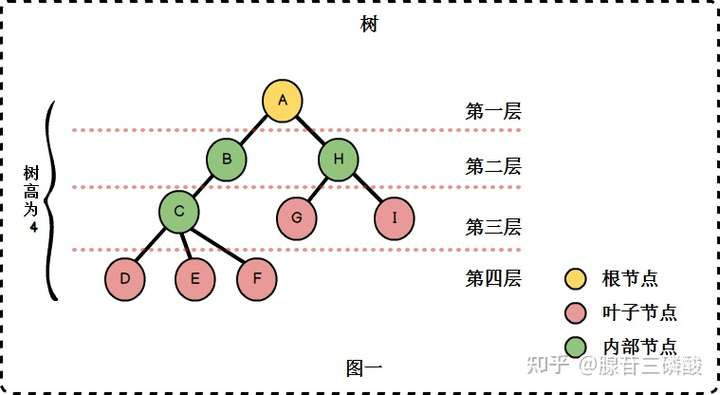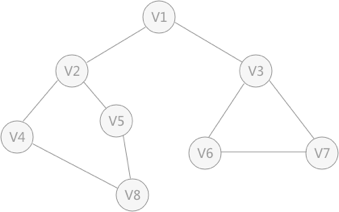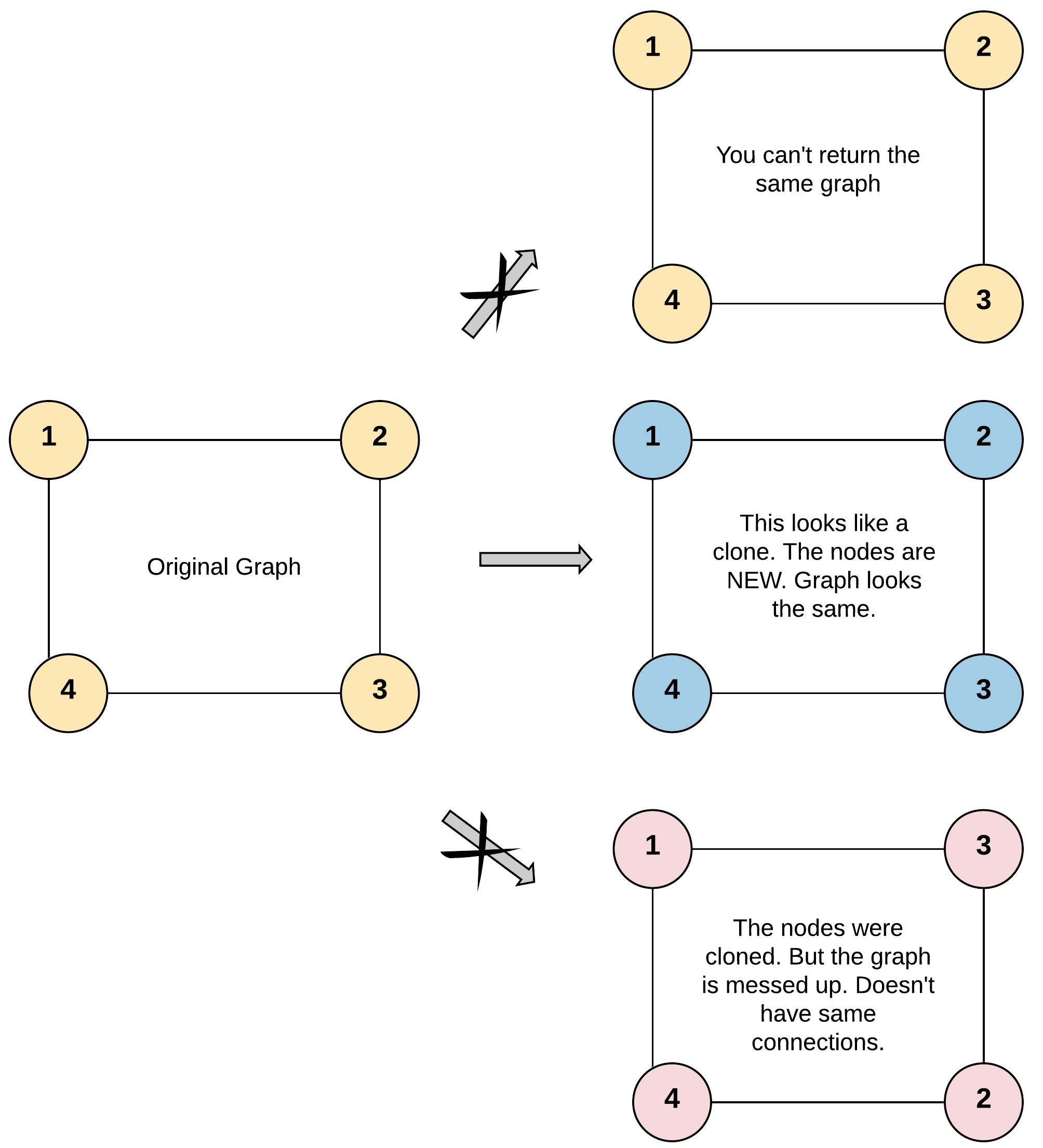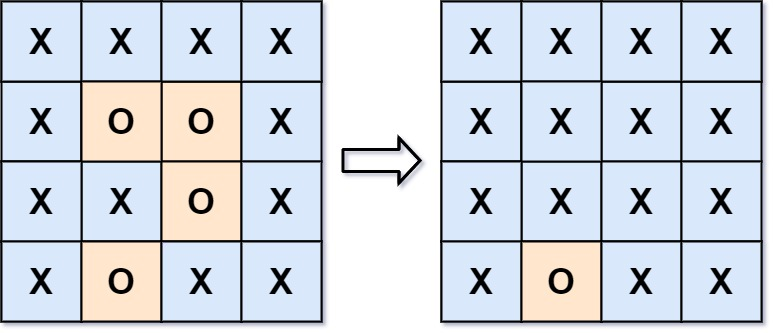# DFS 深度优先搜索的思想

DFS 思修基于递归思想，通过递归的形式来缩小问题规模，把一件事分割成若干个相同的小事，逐步完成。

DFS 有两个重要标志，也就是两个重要数组，一个是标记数组，标记该点是否被访问过，一个用来把该点放入数组，两个点是相辅相承的，通过放入一个起点，并标记起始点，然后依次搜索附近所有能访问的结点，用递归走到下一个点，重复这个步骤，直到走到目标点或是走完全图。## 模板

void dfs()//参数用来表示状态
{
if(到达终点状态)
{
...//根据题意来添加
return;
}

if(越界或者是不符合法状态)//剪枝
return;

for(扩展方式)
{
if(扩展方式所达到状态合法)
{
....//根据题意来添加
标记；
dfs（）；
修改（剪枝）；
(还原标记)；
//是否还原标记根据题意
//如果加上（还原标记）就是 回溯法
}
}
}


## 核心流程• 首先任意找一个未被遍历过的顶点，例如从 V1 开始，由于 V1 率先访问过了，所以，需要标记 V1 的状态为访问过；

• 然后遍历 V1 的邻接点，例如访问 V2 ，并做标记，然后访问 V2 的邻接点，例如 V4 （做标记），然后 V8 ，然后 V5 ；

• 当继续遍历 V5 的邻接点时，根据之前做的标记显示，所有邻接点都被访问过了。此时，从 V5 回退到 V8 ，看 V8 是否有未被访问过的邻接点，如果没有，继续回退到 V4 ， V2 ， V1 ；

• 通过查看 V1 ，找到一个未被访问过的顶点 V3 ，继续遍历，然后访问 V3 邻接点 V6 ，然后 V7 ；

• 由于 V7 没有未被访问的邻接点，所有回退到 V6 ，继续回退至 V3 ，最后到达 V1 ，发现没有未被访问的；

• 最后一步需要判断是否所有顶点都被访问，如果还有没被访问的，以未被访问的顶点为第一个顶点，继续依照上边的方式进行遍历。

V1 -> V2 -> V4 -> V8 -> V5 -> V3 -> V6 -> V7


# 广度优先搜索

V1 -> V2 -> v3 -> V4 -> V5 -> V6 -> V7 -> V8


# 133-克隆图

## 题目

class Node {
public int val;
public List<Node> neighbors;
}


测试用例格式：

• 示例 1：输入：adjList = [[2,4],[1,3],[2,4],[1,3]]



• 节点数不超过 100 。

• 每个节点值 Node.val 都是唯一的，1 <= Node.val <= 100。

• 无向图是一个简单图，这意味着图中没有重复的边，也没有自环。

• 由于图是无向的，如果节点 p 是节点 q 的邻居，那么节点 q 也必须是节点 p 的邻居。

• 图是连通图，你可以从给定节点访问到所有节点。

class Node {
public int val;
public List<Node> neighbors;
public Node() {
val = 0;
neighbors = new ArrayList<Node>();
}
public Node(int _val) {
val = _val;
neighbors = new ArrayList<Node>();
}
public Node(int _val, ArrayList<Node> _neighbors) {
val = _val;
neighbors = _neighbors;
}
}


## 思路1-DFS 深度优先遍历

（1）DFS 深度优先遍历，如何记录被访问过的节点？

（2）如何存放拷贝后的节点

（3）如何深度拷贝

### 深度拷贝

1. 针对节点的拷贝，我们直接创建一个新的 Node(val) 即可。

2. 针对邻居节点的拷贝，我们需要 DFS 创建所有邻居节点的拷贝。

### java 实现

// 定义为 101，直接把 val 当做下标，减少一次减法运算。
Node[] copyArray = new Node;
public Node cloneGraph(Node node) {
//fast-return
if(node == null) {
return null;
}
// 当前节点
// 节点的位置，就是对应的索引的位置。
int val = node.val;
// 如果已经访问，则直接返回已经复制好的节点
if(copyArray[val] != null) {
return copyArray[val];
}
// 拷贝（不拷贝对应的邻居节点）
Node copy = new Node(val, new ArrayList<>(node.neighbors.size()));
copyArray[val] = copy;
// 复制处理邻居节点
for(Node node1 : node.neighbors) {
}
return copy;
}


Runtime: 24 ms, faster than 98.98% of Java online submissions for Clone Graph.
Memory Usage: 38.9 MB, less than 87.79% of Java online submissions for Clone Graph.


### 其他

key是结点类型的指针，那么只有当结点相同时key才会相同，而题目中已经指明了每个结点的val是唯一的，所以val相同一定可以推出结点相同，进而推出Key相同

## 思路2-BFS 广度优先遍历

### 实现流程

（1）存放当前 node 到 stack 中，拷贝对象放到 copyArray 中。

（2）遍历 stack

（3）返回 copyArray[node.val]

### java 实现

Node[] copyArray = new Node;

public Node cloneGraph(Node node) {
//fast-return
if(node == null) {
return null;
}
// 当前节点
// 节点的位置，就是对应的索引的位置。
Stack<Node> stack = new Stack<>();
stack.push(node);
// 克隆第一个节点并存储到哈希表中
int val = node.val;
copyArray[val]  = new Node(val, new ArrayList<>());
while (!stack.isEmpty()) {
Node pop = stack.pop();
// 处理邻居节点
for(Node neighbor : pop.neighbors) {
// 复制邻居节点
int nVal = neighbor.val;
Node copy = copyArray[nVal];
// 没有访问过
if(copy == null) {
copy = new Node(nVal, new ArrayList<>());
copyArray[nVal] = copy;
stack.push(neighbor);
}
// 更新拷贝的邻居节点
}
}
// 直接获取拷贝后的 node
return copyArray[val];
}


Runtime: 25 ms, faster than 90.62% of Java online submissions for Clone Graph.
Memory Usage: 39.1 MB, less than 66.44% of Java online submissions for Clone Graph.


# 应用实战篇

## 题目

130. 被围绕的区域输入：board = [["X","X","X","X"],["X","O","O","X"],["X","X","O","X"],["X","O","X","X"]]



输入：board = [["X"]]



m == board.length

n == board[i].length

1 <= m, n <= 200

board[i][j] 为 ‘X’ 或 ‘O’

## 思路1：DFS

（1）任何边界上的 ’O’ 都不会被填充为 ’X’

（2）任何不在边界上，或不与边界上的 ’O’ 相连的 ’O’ 最终都会被填充为 ’X’。

### java 实现

private int m;
private int n;
public void solve(char[][] board) {
m = board.length;
n = board.length;
if(m <= 2 || n <= 2) {
return;
}
// 第一行和最后一行
for(int i = 0; i < n; i++) {
dfs(board, 0, i);
dfs(board, m-1, i);
}
// 第一列和最后一列
for(int i = 1; i < m-1; i++) {
dfs(board, i, 0);
dfs(board, i, n-1);
}
// 统一处理
for(int i = 0; i < m; i++) {
for(int j = 0; j < n; j++) {
if(board[i][j] == '#') {
board[i][j] = 'O';
} else if(board[i][j] == 'O') {
board[i][j] = 'X';
}
}
}
}

private void dfs(char[][] board, int i, int j) {
if(i < 0 || i >= m || j < 0 || j >= n || board[i][j] != 'O') {
return;
}
// 标记
board[i][j] = '#';
dfs(board, i+1, j);
dfs(board, i-1, j);
dfs(board, i, j+1);
dfs(board, i, j-1);
}


### 效果

Runtime: 1 ms, faster than 99.63% of Java online submissions for Surrounded Regions.
Memory Usage: 41 MB, less than 66.64% of Java online submissions for Surrounded Regions.


## dsf 非递归:

class Solution {
public class Pos{
int i;
int j;
Pos(int i, int j) {
this.i = i;
this.j = j;
}
}

public void solve(char[][] board) {
if (board == null || board.length == 0) return;
int m = board.length;
int n = board.length;
for (int i = 0; i < m; i++) {
for (int j = 0; j < n; j++) {
// 从边缘第一个是o的开始搜索
boolean isEdge = i == 0 || j == 0 || i == m - 1 || j == n - 1;
if (isEdge && board[i][j] == 'O') {
dfs(board, i, j);
}
}
}

for (int i = 0; i < m; i++) {
for (int j = 0; j < n; j++) {
if (board[i][j] == 'O') {
board[i][j] = 'X';
}
if (board[i][j] == '#') {
board[i][j] = 'O';
}
}
}
}

public void dfs(char[][] board, int i, int j) {
Stack<Pos> stack = new Stack<>();
stack.push(new Pos(i, j));
board[i][j] = '#';
while (!stack.isEmpty()) {
// 取出当前stack 顶, 不弹出.
Pos current = stack.peek();
// 上
if (current.i - 1 >= 0
&& board[current.i - 1][current.j] == 'O') {
stack.push(new Pos(current.i - 1, current.j));
board[current.i - 1][current.j] = '#';
continue;
}
// 下
if (current.i + 1 <= board.length - 1
&& board[current.i + 1][current.j] == 'O') {
stack.push(new Pos(current.i + 1, current.j));
board[current.i + 1][current.j] = '#';
continue;
}
// 左
if (current.j - 1 >= 0
&& board[current.i][current.j - 1] == 'O') {
stack.push(new Pos(current.i, current.j - 1));
board[current.i][current.j - 1] = '#';
continue;
}
// 右
if (current.j + 1 <= board.length - 1
&& board[current.i][current.j + 1] == 'O') {
stack.push(new Pos(current.i, current.j + 1));
board[current.i][current.j + 1] = '#';
continue;
}
// 如果上下左右都搜索不到,本次搜索结束，弹出stack
stack.pop();
}
}
}


## bfs 非递归:

dfs 非递归的时候我们用 stack 来记录状态，而 bfs 非递归，我们则用队列来记录状态。

class Solution {
public class Pos{
int i;
int j;
Pos(int i, int j) {
this.i = i;
this.j = j;
}
}
public void solve(char[][] board) {
if (board == null || board.length == 0) return;
int m = board.length;
int n = board.length;
for (int i = 0; i < m; i++) {
for (int j = 0; j < n; j++) {
// 从边缘第一个是o的开始搜索
boolean isEdge = i == 0 || j == 0 || i == m - 1 || j == n - 1;
if (isEdge && board[i][j] == 'O') {
bfs(board, i, j);
}
}
}

for (int i = 0; i < m; i++) {
for (int j = 0; j < n; j++) {
if (board[i][j] == 'O') {
board[i][j] = 'X';
}
if (board[i][j] == '#') {
board[i][j] = 'O';
}
}
}
}

public void bfs(char[][] board, int i, int j) {
board[i][j] = '#';
while (!queue.isEmpty()) {
Pos current = queue.poll();
// 上
if (current.i - 1 >= 0
&& board[current.i - 1][current.j] == 'O') {
board[current.i - 1][current.j] = '#';
// 没有continue.
}
// 下
if (current.i + 1 <= board.length - 1
&& board[current.i + 1][current.j] == 'O') {
board[current.i + 1][current.j] = '#';
}
// 左
if (current.j - 1 >= 0
&& board[current.i][current.j - 1] == 'O') {
board[current.i][current.j - 1] = '#';
}
// 右
if (current.j + 1 <= board.length - 1
&& board[current.i][current.j + 1] == 'O') {
board[current.i][current.j + 1] = '#';
}
}
}
}


## 并查集:

find(int m)：这是并查集的基本操作，查找 mm 的根节点。

isConnected(int m,int n)：判断 m，nm，n 两个点是否在一个连通区域。

union(int m,int n):合并 m，nm，n 两个点所在的连通区域。

class UnionFind {
int[] parents;

public UnionFind(int totalNodes) {
parents = new int[totalNodes];
for (int i = 0; i < totalNodes; i++) {
parents[i] = i;
}
}
// 合并连通区域是通过find来操作的, 即看这两个节点是不是在一个连通区域内.
void union(int node1, int node2) {
int root1 = find(node1);
int root2 = find(node2);
if (root1 != root2) {
parents[root2] = root1;
}
}

int find(int node) {
while (parents[node] != node) {
// 当前节点的父节点 指向父节点的父节点.
// 保证一个连通区域最终的parents只有一个.
parents[node] = parents[parents[node]];
node = parents[node];
}

return node;
}

boolean isConnected(int node1, int node2) {
return find(node1) == find(node2);
}
}


1. 和边界上的 OO 在一个连通区域内的。这些 OO 我们保留。

2. 不和边界上的 OO 在一个连通区域内的。这些 OO 就是被包围的，替换。

public void solve(char[][] board) {
if (board == null || board.length == 0)
return;

int rows = board.length;
int cols = board.length;

// 用一个虚拟节点, 边界上的O 的父节点都是这个虚拟节点
UnionFind uf = new UnionFind(rows * cols + 1);
int dummyNode = rows * cols;

for (int i = 0; i < rows; i++) {
for (int j = 0; j < cols; j++) {
if (board[i][j] == 'O') {
// 遇到O进行并查集操作合并
if (i == 0 || i == rows - 1 || j == 0 || j == cols - 1) {
// 边界上的O,把它和dummyNode 合并成一个连通区域.
uf.union(node(i, j), dummyNode);
} else {
// 和上下左右合并成一个连通区域.
if (i > 0 && board[i - 1][j] == 'O')
uf.union(node(i, j), node(i - 1, j));
if (i < rows - 1 && board[i + 1][j] == 'O')
uf.union(node(i, j), node(i + 1, j));
if (j > 0 && board[i][j - 1] == 'O')
uf.union(node(i, j), node(i, j - 1));
if (j < cols - 1 && board[i][j + 1] == 'O')
uf.union(node(i, j), node(i, j + 1));
}
}
}
}

for (int i = 0; i < rows; i++) {
for (int j = 0; j < cols; j++) {
if (uf.isConnected(node(i, j), dummyNode)) {
// 和dummyNode 在一个连通区域的,那么就是O；
board[i][j] = 'O';
} else {
board[i][j] = 'X';
}
}
}
}

int node(int i, int j) {
return i * cols + j;
}
}


# NEXT

Number of Islands

Walls and Gates

# 参考资料

https://leetcode-cn.com/problems/surrounded-regions/solution/bfsdi-gui-dfsfei-di-gui-dfsbing-cha-ji-by-ac_pipe/

https://www.guyuehome.com/11046

https://lucifer.ren/leetcode/thinkings/DFS.html

https://developer.aliyun.com/article/49140

https://blog.csdn.net/weixin_42638946/article/details/114993465

https://blog.csdn.net/qq_40310148/article/details/106786652